﻿ 流激围壳结构的低频噪声数值模拟研究
 舰船科学技术2016, Vol. 38Issue (6): 154-157PDF

1. 92512部队, 辽宁 大连 116013 ;
2. 大连测控技术研究所, 辽宁 大连 116013

Numerical modeling on submarine sail flow-induced noise within low frequency
Xu Ji-bo1, Guo He-ping2, Wang Zhi-wei2
1. No. 92512 Unit of PLA, Dalian 116013, China ;
2. Dalian Scientific Test and Control Technology Institute, Dalian 116013, China
Abstract: The sail is the most important and conspicuous structure of a submarine, the noise level of which may strongly influence the usage of the sub. Numerical modeling on flow-induce noise to the sail was done towards several diverse circumstances based on FEM and BEM methods, the results indicate that differences do exist within different voyage ways and different structures, evidences are following provided for further sail design and practical operation.
Key words: Sail     Flow-induced noise     Numerical modeling
0 引 言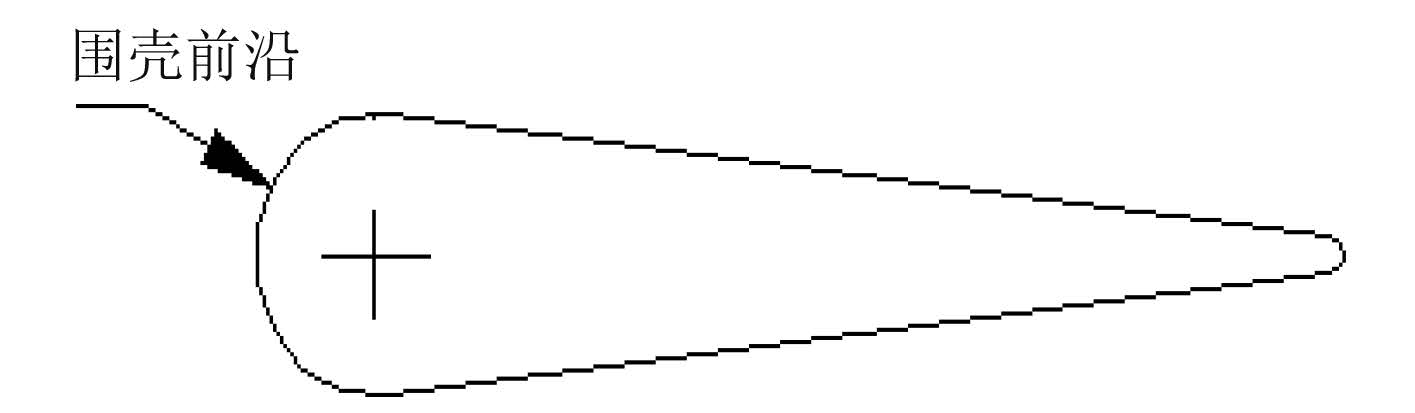图 1 围壳垂向 CAD 效果 Fig. 1 Vertical result effect of the sail

1 围壳结构模态分析

 ${ M}\mathop \delta \limits^{..} + { C}\mathop \delta \limits^. + { K}\delta = { F}\text{。}$ (1)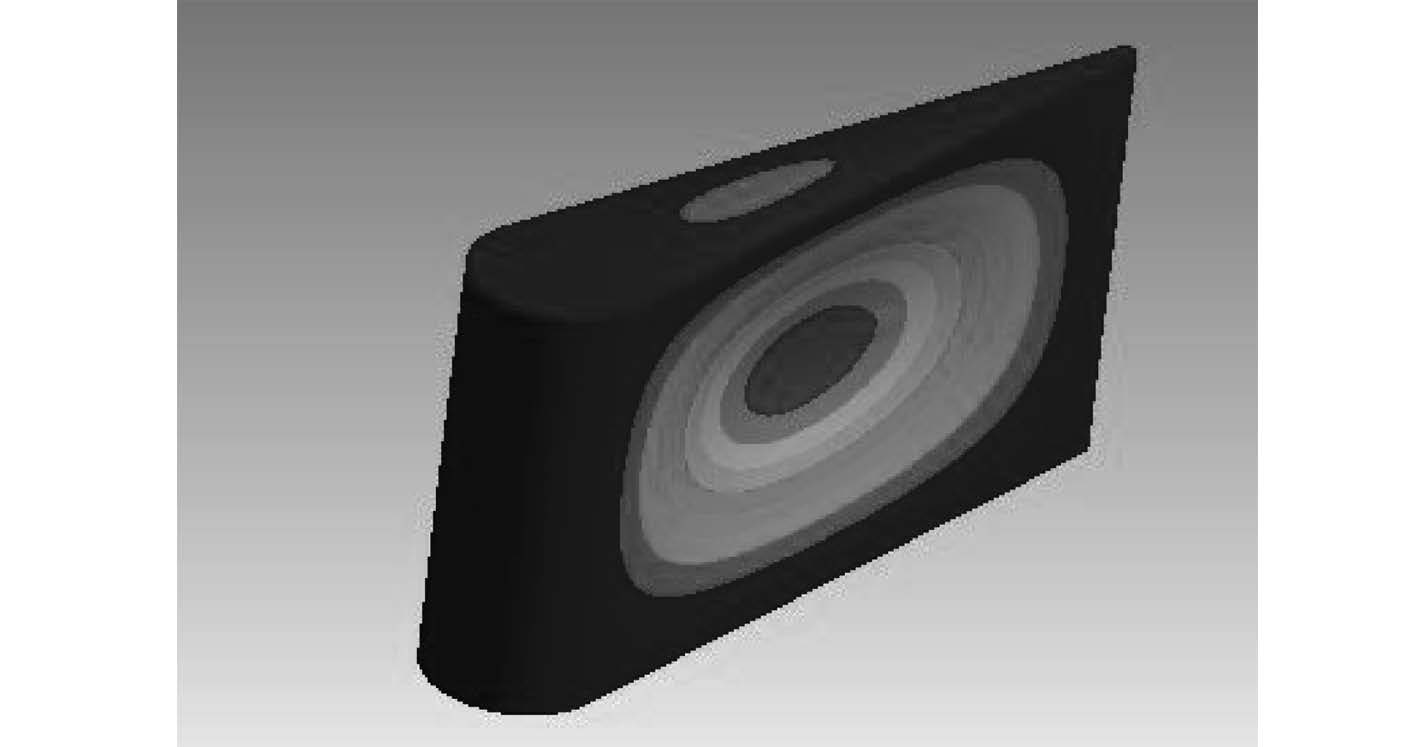图 2 初始结构第 1 阶阵型 Fig. 2 First formation of original structure
2 流场脉动压力及动力学响应数值计算

κ-ε 模型在系统方程里引入了 2 个新变量。流体连续方程为：

 $\frac{{\partial \rho }}{{\partial t}} + \nabla \cdot \left( {\rho {{U}}} \right) = 0\text{，}$ (2)

 $\frac{{\partial \rho {{U}}}}{{\partial t}} \!+\! \nabla \cdot \left( {\rho {{U*U}}} \right) \!-\! \nabla \cdot \left( {{\mu _{{\rm{eff}}}}\nabla {{U}}} \right) \!=\! \nabla \cdot {\rm{p' \!+\! }}\nabla \cdot {\left( {{\mu _{{\rm{eff}}}}\nabla {{U}}} \right)^{\rm{T}}} \!+\! {\rm{B}}\text{，}$ (3)

 ${\mu _{{\rm{eff}}}}{\rm{ = }}\mu + {{{C}}_\mu }\rho \frac{{{{{K}}^2}}}{\varepsilon }\text{，}$ (4)

kε 的值可以通过湍流动能方程求解，湍流动能方程和耗散率方程分别为

 $\begin{array}{l} \displaystyle\frac{{\partial \left( {\rho {\rm{k}}} \right)}}{{\partial t}} \!+\! \nabla \cdot \left( {\rho {{U_k}}} \right) \!=\! \nabla \cdot \left[{\left( {\mu \!+\! \frac{{{\mu _{\rm{t}}}}}{{{\sigma _{\rm{k}}}}}} \right)\nabla {{k}}} \right] + {{{P}}_{{k}}} - \rho \varepsilon \text{，}\\ \displaystyle\frac{{\partial \left( {\rho \varepsilon } \right)}}{{\partial t}}\!\!+\!\! \nabla \!\!\cdot\!\! \left( {\rho {{U}}_\varepsilon } \right) \!\!=\! \!\nabla \!\!\cdot\!\! \left[{\left( {\mu \!\!\!+\!\! \frac{{{\mu _{\rm{t}}}}}{{{\sigma _\varepsilon }}}} \right)\!\!\nabla \varepsilon } \right] \!\!+\!\! \frac{\varepsilon }{{{k}}}\left( {{{{C}}_{{\varepsilon _1}}}{{{P}}_{{k}}} \!\!-\!\! {{{C}}_{{\varepsilon _2}}}\rho \varepsilon } \right)\text{。} \end{array}$ (5)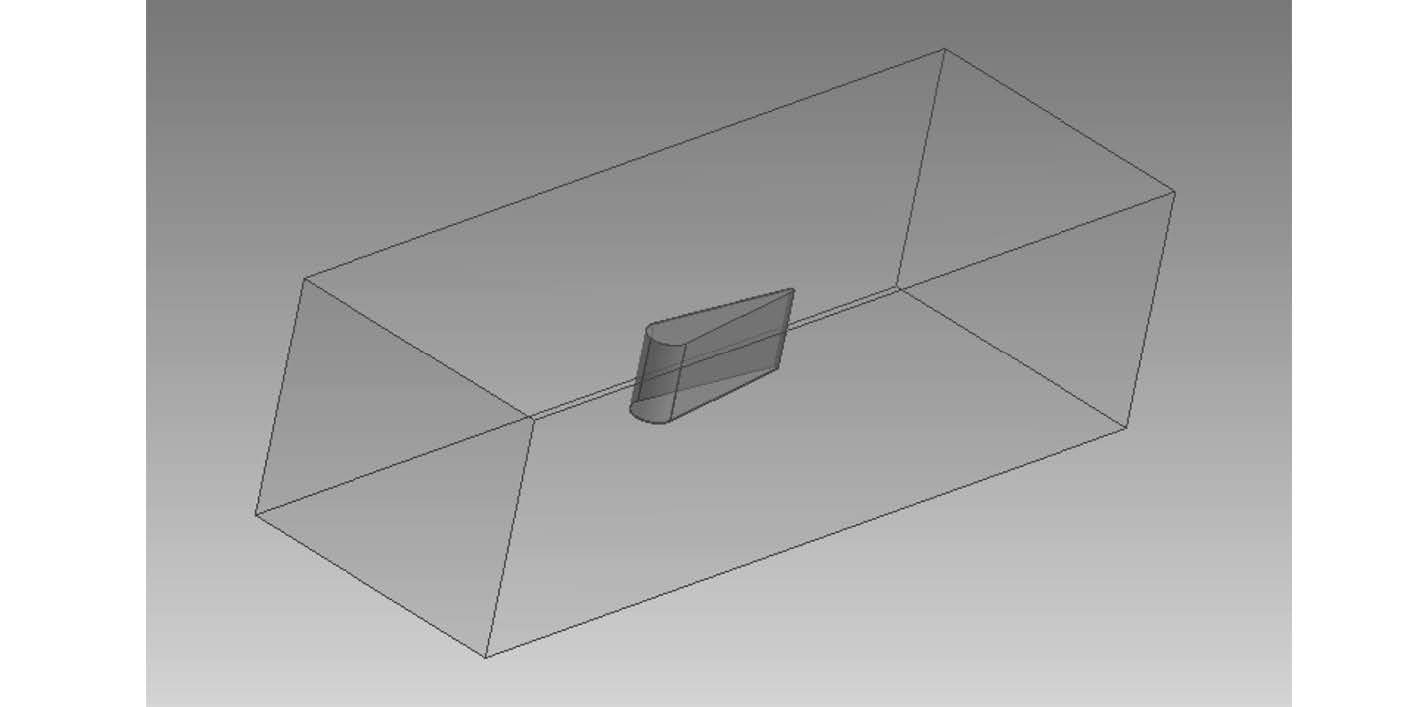图 3 流场搭建效果 Fig. 3 luid model effect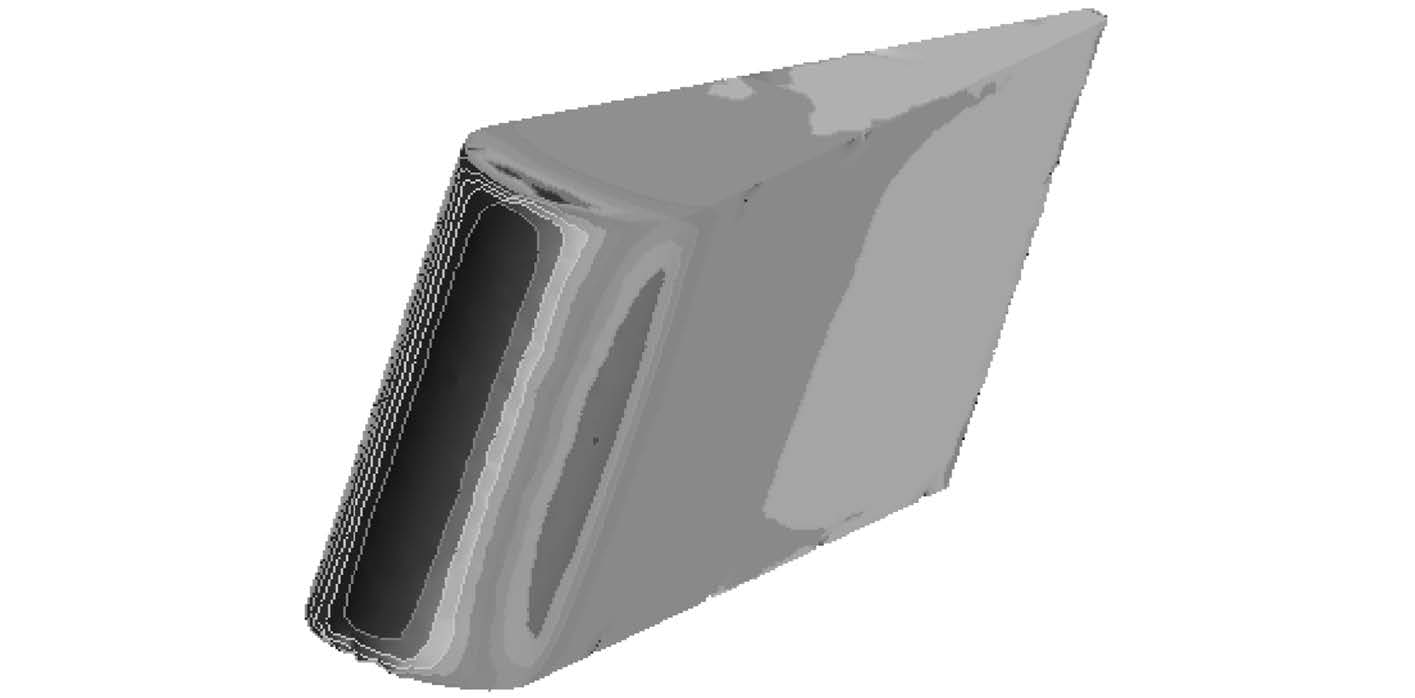图 4 围壳压力脉动云图 Fig. 4 Cloud picture of pressure pulsation over the sail

3 流激噪声数值计算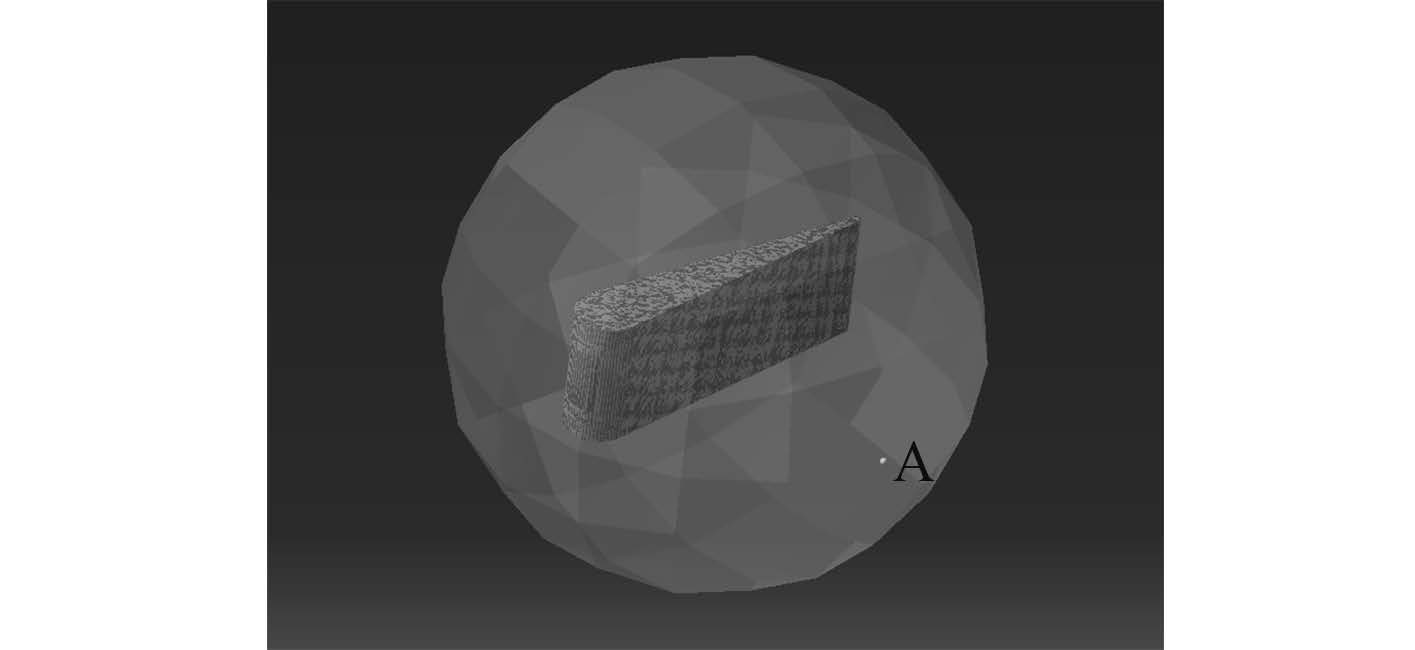图 5 边界元网格及声场建模效果 Fig. 5 BEM mesh and the acoustic model effect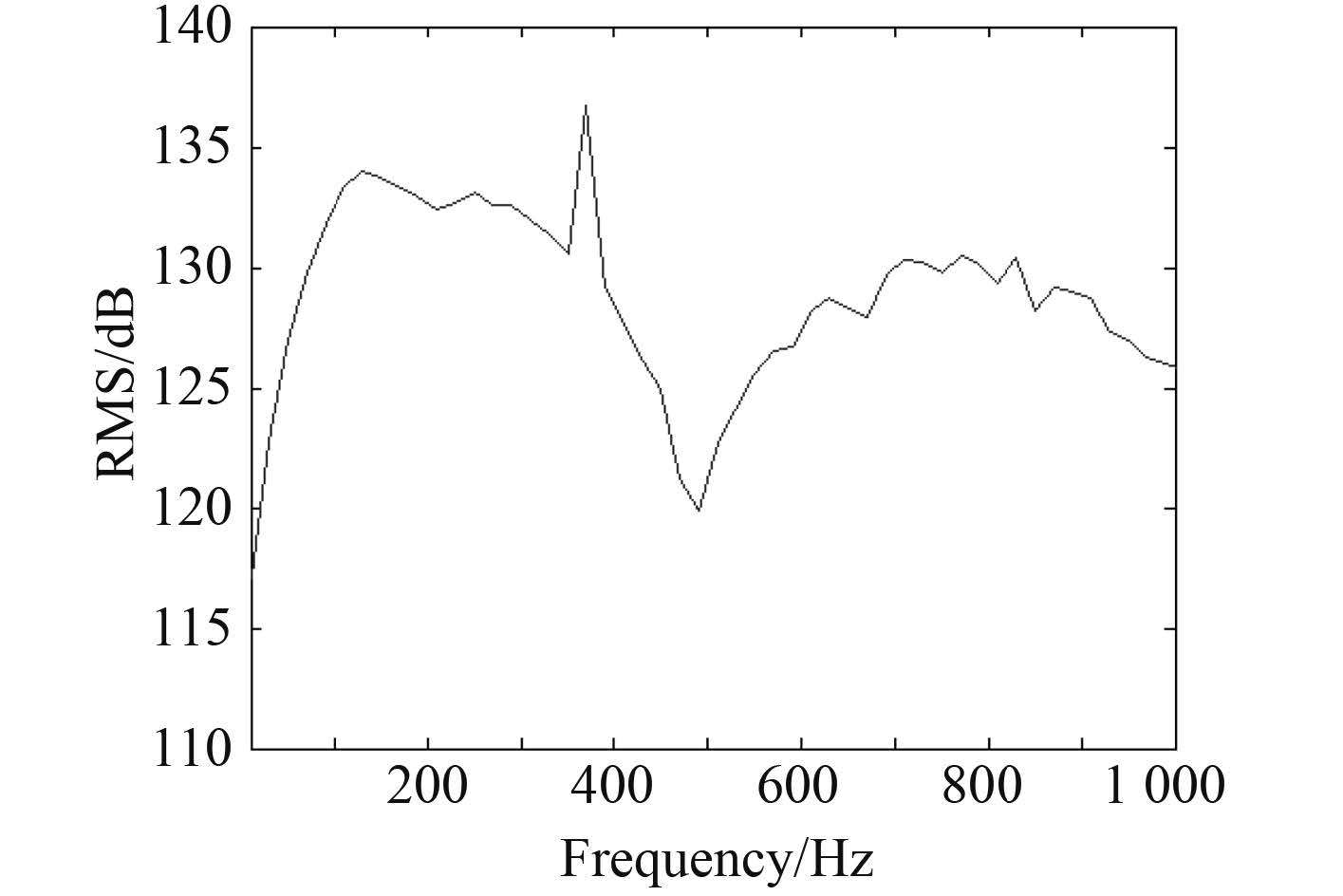图 6 初始 6 kn 测点声压级 Fig. 6 SPL of the specific measure point with original 6 knots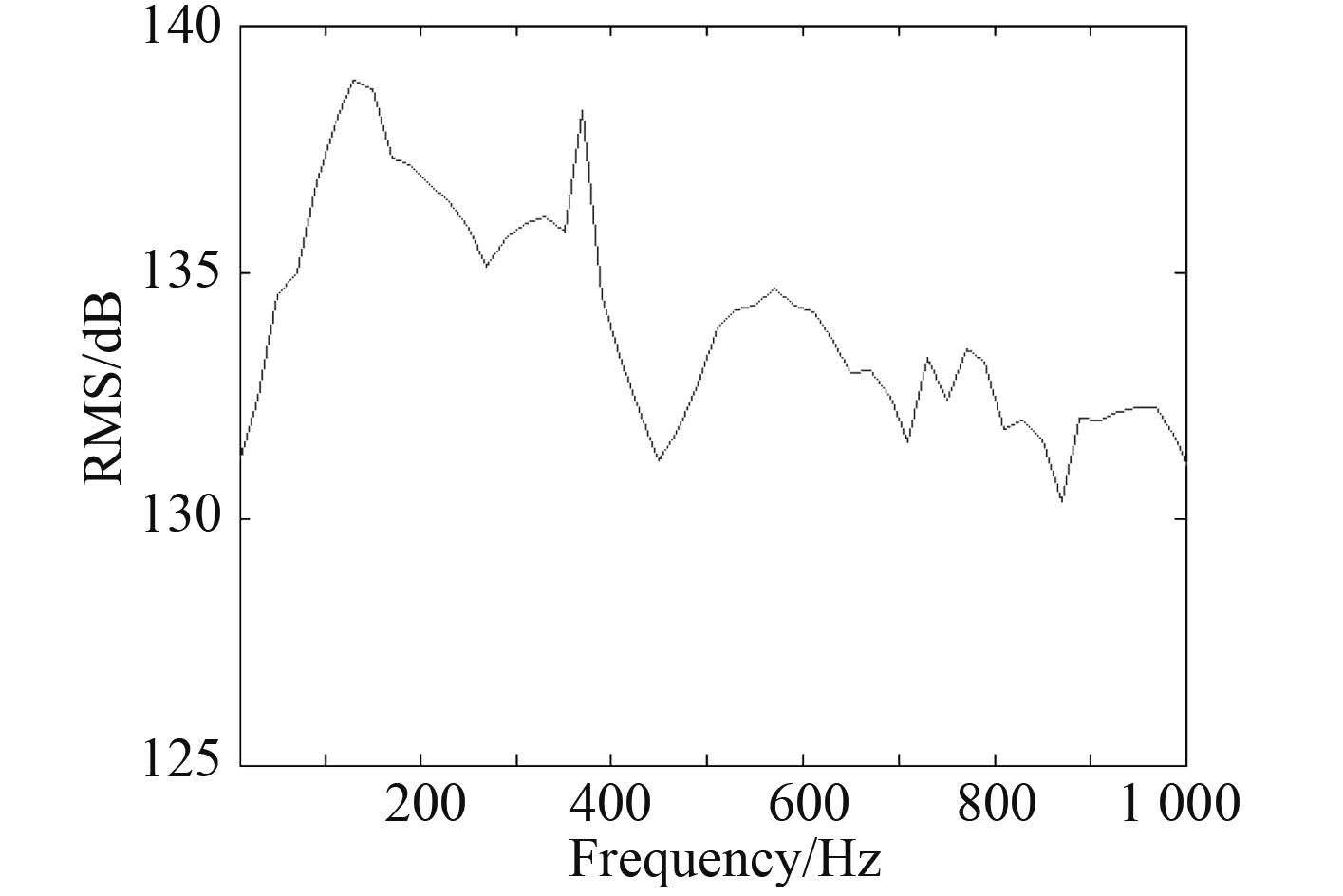图 7 增速至 8 kn 测点声压级 Fig. 7 SPL of the specific measure point with speeding up to 8 knots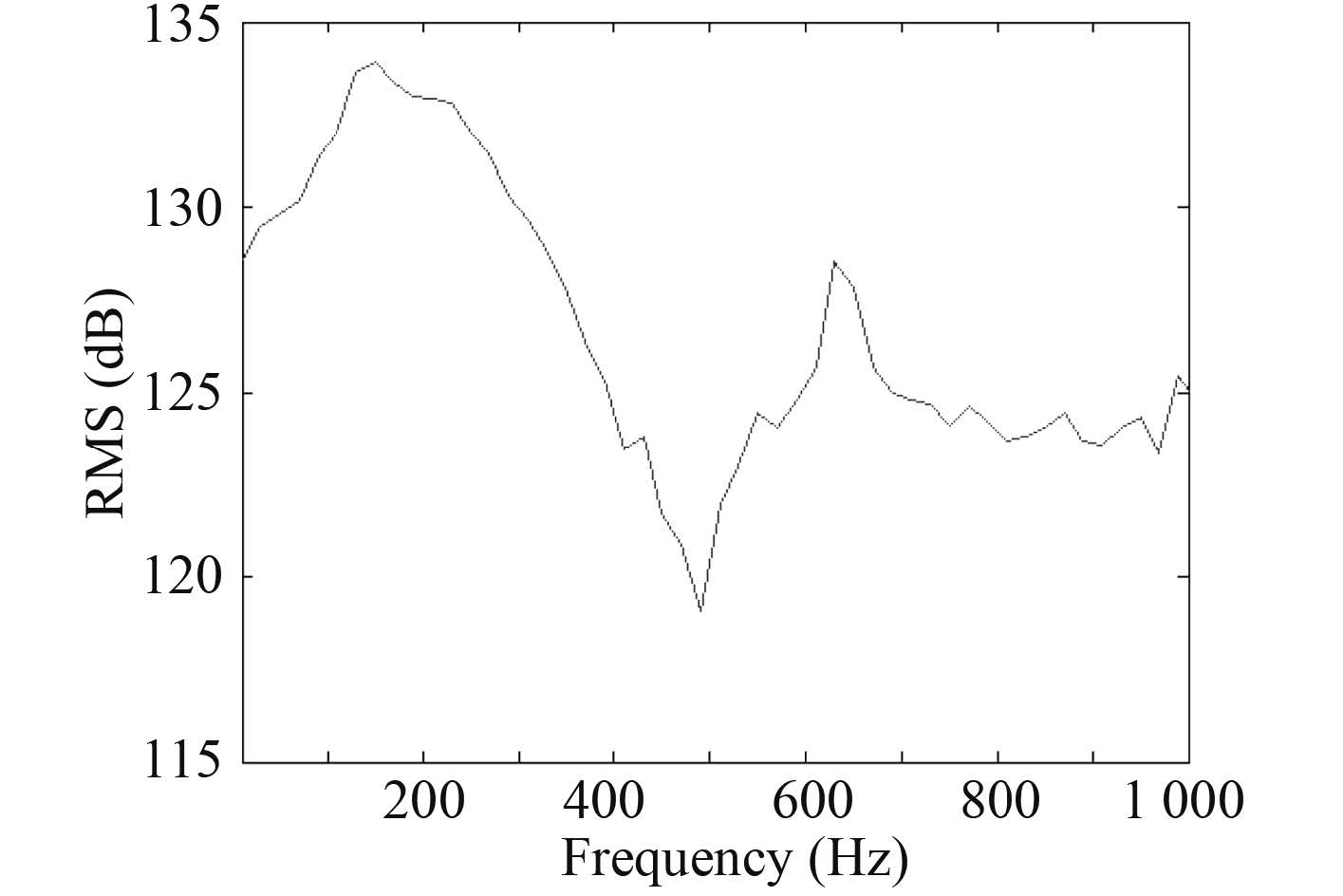图 8 增高 2 m 测点声压级 Fig. 8 SPL of the specific measure point with height increasing 2 meters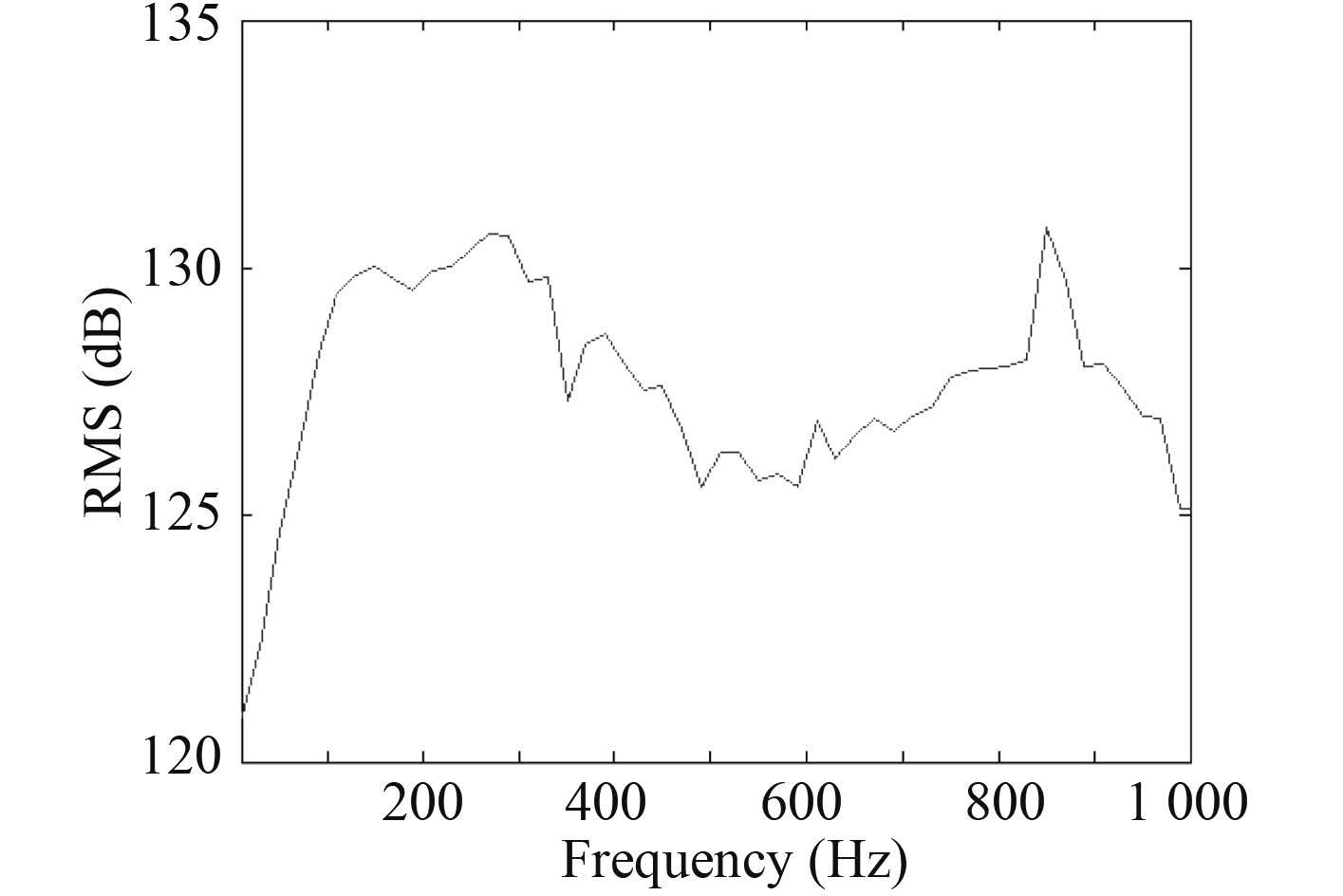图 9 增长 2 m 节测点声压级 Fig. 9 SPL of the specific measure point with length increasing to 2 meters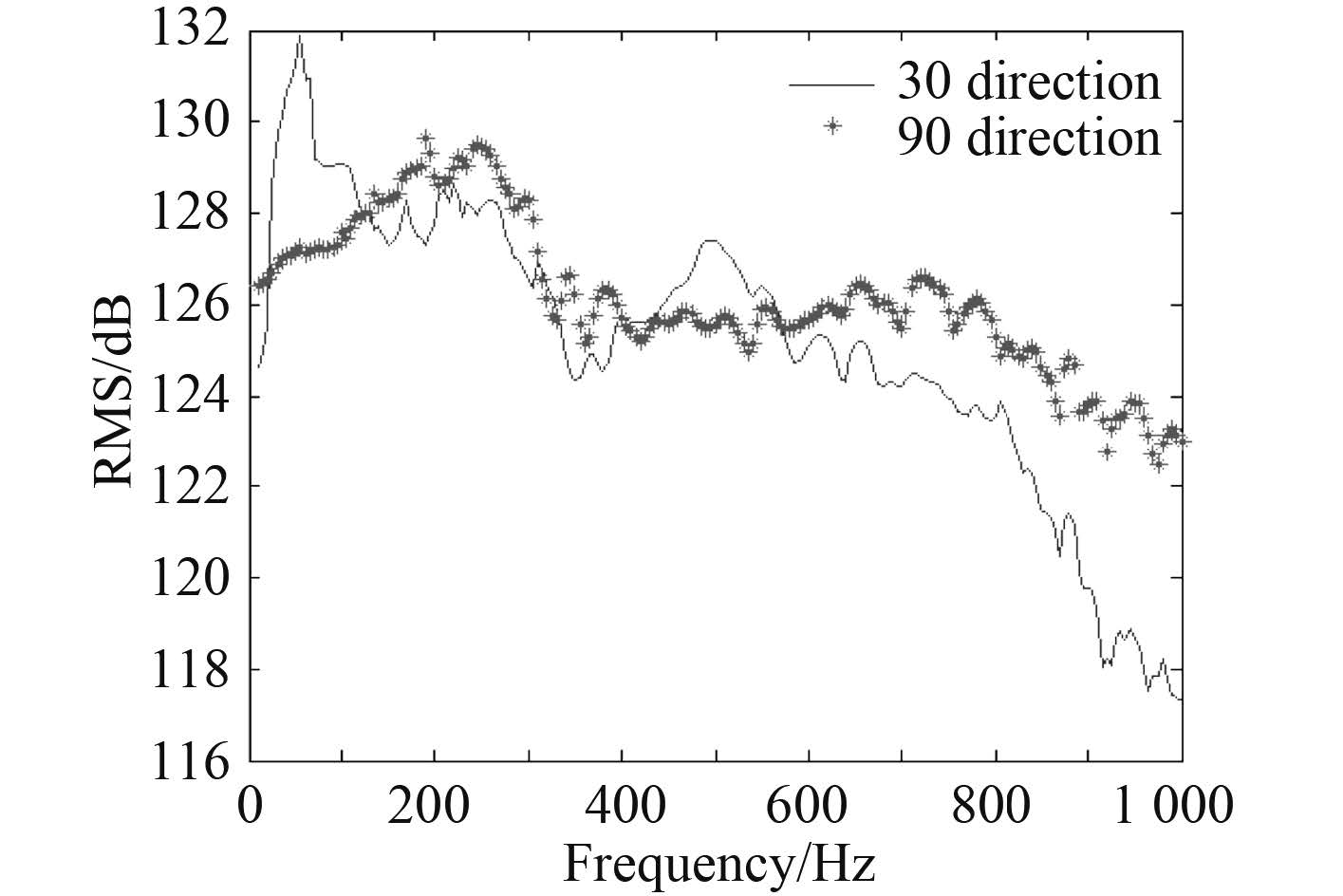图 10 不同流向条件下的测点声压级 Fig. 10 SPL of the different flow conditions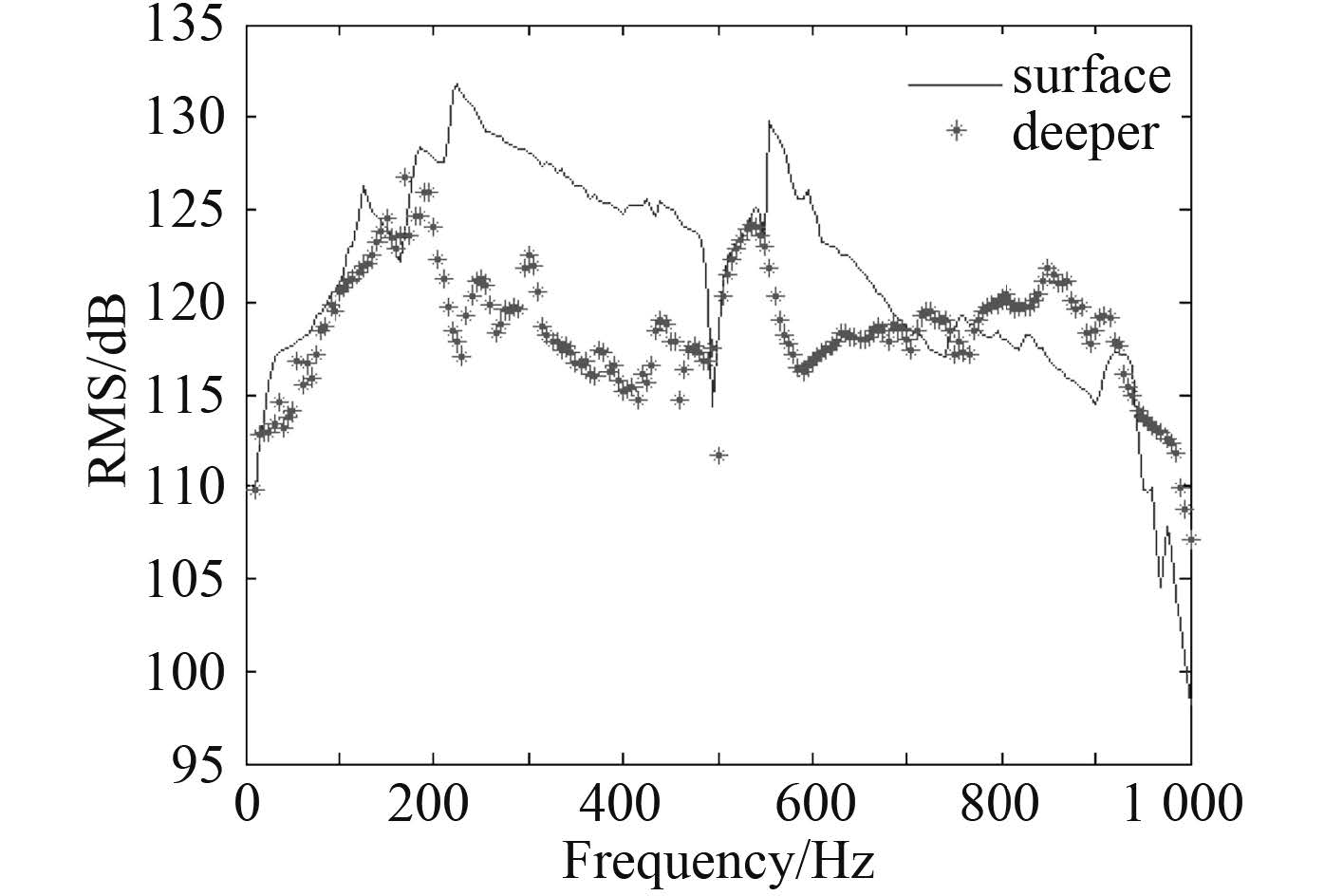图 11 不同深度下的测点声压级 Fig. 11 SPL of the different depth conditions
4 结 语

  侯本龙. 围壳结构流激振动噪声特性研究[D]. 哈尔滨:哈尔滨工程大学, 2011.  聂毅, 余雄庆. 翼面隐身结构优化设计[J]. 南京航空航天大学学报 , 2008, 40 (4) :465–468.  郑杨. 围壳结构声辐射机理研究[D]. 哈尔滨:哈尔滨工程大学, 2009.  江文成. 潜艇流噪声与流固耦合作用下流激噪声的数值模拟[D]. 上海:上海交通大学, 2013.  OLSON L G, BATHE K J. A study of displacement-based fluid finite elements for calculating frequencies of fluid and fluid-structure systems[J]. Nuclear engineering and design , 1983, 76 (2) :137–151. DOI:10.1016/0029-5493(83)90130-9  PETYT M, LEA J, KOOPMANN G H. A finite element method for determining the acoustic modes of irregular shaped cavities[J]. Journal of sound and vibration , 1976, 45 (4) :495–502. DOI:10.1016/0022-460X(76)90730-6  王福军. 计算流体力学分析:CFD软件原理与应用[M]. 北京: 清华大学出版社, 2004 .  SANDBERG G, GÖRANSSON P. A symmetric finite element formulation for acoustic fluid-structure interaction analysis[J]. Journal of sound and vibration , 1988, 123 (3) :507–515. DOI:10.1016/S0022-460X(88)80166-4  杜波, 黄建伟, 陈源. 潜艇指挥室围壳顶部型线构型[J]. 船海工程 , 2007, 36 (2) :107–110.  BREBBIA C A. The boundary element method for engineers[M]. New York: Halstead Press, 1978 .